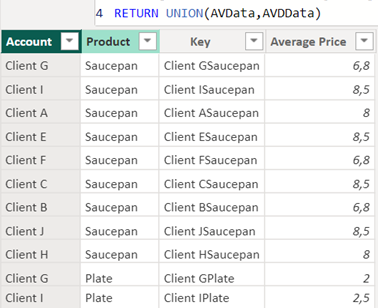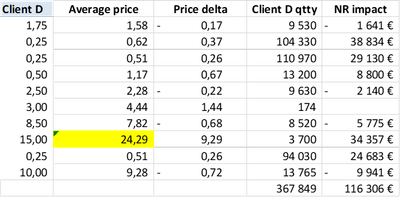cancel
Showing results for
Did you mean:

Fabric is Generally Available. Browse Fabric Presentations. Work towards your Fabric certification with the Cloud Skills Challenge.Frequent Visitor

## A measure (NR impact) based on measures from two different tables

Hello everyone,

I need to calculate a Net Revenue impact, based on measures calculated in two different tables.

1. I have a calculated table, that consists of Account (Customer), Product, and Average Price:Based on this table I calculated:

1. the average of average price (like in Pivot table) for all clients, except for Client D

= CALCULATE(AVERAGE('Average of Contract price'[Average Price]),'Average of Contract price'[Account]<>"Client D")

2. the average of average price for Client D

= CALCULATE(AVERAGE('Average of Contract price'[Average Price]),'Average of Contract price'[Account]="Client D")

3. Price Delta (Avg. price for Client D - Avg. price for the rest of the clients)

= 'Average of Contract price'[AveragePrice2]-'Average of Contract price'[AveragePrice1]

The other table contains quantities for Client D (column Volume):I created a simple measure that sums the quantities for Client D.

What I need is to multiply the Price Delta (the third masure) with the SUM of the quantities for client D in order to get the Net Revenue impact.

1 ACCEPTED SOLUTIONMemorable Member

I see that you want to calculate the Price Delta and the Net Revenue impact per product and not aggregate it across all customers/dates. To achieve this, you need to use context transition functions in DAX to perform the calculations at the product level. You can modify your measures as follows:

Assuming you have a product identifier (let's call it "ProductID") in your tables, you can create the following measures:

1. Price Delta per Product:

Price Delta per Product =
SUMX(
FILTER('Average of Contract price', 'Average of Contract price'[Account] = "Client D"),
'Average of Contract price'[AveragePrice2] - 'Average of Contract price'[AveragePrice1]
)

This measure calculates the Price Delta for each product separately by iterating over the 'Average of Contract price' table and applying a filter for "Client D."

1. Total Quantity per Product for Client D (assuming you have a 'Volume' table with a relationship to your product table):

Total Quantity per Product Client D =
SUMX(
FILTER('Volume', 'Volume'[Customer] = "Client D"),
'Volume'[Volume]
)

This measure calculates the total quantity for Client D per product.

1. Net Revenue Impact per Product:

Net Revenue Impact per Product = [Price Delta per Product] * [Total Quantity per Product Client D]

Now, when you use the "Net Revenue Impact per Product" measure in your visuals, it should correctly calculate the impact at the product level, as shown in your Excel example.

8 REPLIES 8Super User

@LStoychev Great you have the solution, the only thing I will add is that you don't need to create a calculated table for this calculation. If the purpose of that table is just to go net impact then it can be done using a measure without a calculated table—just my 2 cents.

Subscribe to the @PowerBIHowTo YT channel for an upcoming video on List and Record functions in Power Query!!

Learn Power BI and Fabric - subscribe to our YT channel - Click here: @PowerBIHowTo

If my solution proved useful, I'd be delighted to receive Kudos. When you put effort into asking a question, it's equally thoughtful to acknowledge and give Kudos to the individual who helped you solve the problem. It's a small gesture that shows appreciation and encouragement! ❤

Did I answer your question? Mark my post as a solution. Proud to be a Super User! Appreciate your Kudos 🙂
Feel free to email me with any of your BI needs.Super User

@LStoychev I don't think you need to create a calculated table to achieve the goal. It will be easier if you can share pbix file with sample data with the expected output and I will get you the solution. You can share using one drive/google drive.

Subscribe to the @PowerBIHowTo YT channel for an upcoming video on List and Record functions in Power Query!!

Learn Power BI and Fabric - subscribe to our YT channel - Click here: @PowerBIHowTo

If my solution proved useful, I'd be delighted to receive Kudos. When you put effort into asking a question, it's equally thoughtful to acknowledge and give Kudos to the individual who helped you solve the problem. It's a small gesture that shows appreciation and encouragement! ❤

Did I answer your question? Mark my post as a solution. Proud to be a Super User! Appreciate your Kudos 🙂
Feel free to email me with any of your BI needs.Frequent Visitor

Thanks Parry2k,

You can find below 2 links. The first one is for the PBI dashboard, the second one contains a sample data.

I wanted to calculate the avg. of the avg. product prices for all customers, except for Client D. - I have already done this (see the measure AveragePrice2, used in the first visual).

Then I subtracted from it the average prices of Client D (and I got the Price Delta for all products).

What I want to do now, is to multiply the Client D's volume by the Price Delta (in order to get the NR impact).

The NR impact that you see in the second visual is calculated in Excel, not in PBI. I want to calculate it, using PBI.

Thanks again.Memorable Member

To calculate the Net Revenue impact by multiplying the Price Delta with the SUM of quantities for Client D, you can create a new measure in your Power BI report. Based on the measures and tables you provided, here's how you can create this new measure:

Assuming your calculated table with the Price Delta is called "Average of Contract price," and the table with quantities for Client D is called something like "Client D Quantities," you can create a measure as follows:

Net Revenue Impact =
VAR PriceDelta = 'Average of Contract price'[AveragePrice2] - 'Average of Contract price'[AveragePrice1]
VAR ClientDQuantity = SUM('Client D Quantities'[Volume])
RETURN
PriceDelta * ClientDQuantity

This DAX measure first calculates the Price Delta using the Price Delta formula you provided. Then, it calculates the SUM of quantities for Client D from the "Client D Quantities" table. Finally, it returns the product of PriceDelta and ClientDQuantity, which gives you the Net Revenue impact you're looking for.

You can add this measure to your report, and it should provide you with the Net Revenue impact based on the measures from the two different tables.Frequent Visitor

Hi 123abc, thank you for responding so quickly.

The problem is that using this measure, PBI performs the calculation multiple times (for all customers/dates), instead of just once per product, and I get a wrong result in the visual:I have calculated the same thing in Excel, and that is the result I am trying to achieve:Memorable Member

I see that you want to calculate the Price Delta and the Net Revenue impact per product and not aggregate it across all customers/dates. To achieve this, you need to use context transition functions in DAX to perform the calculations at the product level. You can modify your measures as follows:

Assuming you have a product identifier (let's call it "ProductID") in your tables, you can create the following measures:

1. Price Delta per Product:

Price Delta per Product =
SUMX(
FILTER('Average of Contract price', 'Average of Contract price'[Account] = "Client D"),
'Average of Contract price'[AveragePrice2] - 'Average of Contract price'[AveragePrice1]
)

This measure calculates the Price Delta for each product separately by iterating over the 'Average of Contract price' table and applying a filter for "Client D."

1. Total Quantity per Product for Client D (assuming you have a 'Volume' table with a relationship to your product table):

Total Quantity per Product Client D =
SUMX(
FILTER('Volume', 'Volume'[Customer] = "Client D"),
'Volume'[Volume]
)

This measure calculates the total quantity for Client D per product.

1. Net Revenue Impact per Product:

Net Revenue Impact per Product = [Price Delta per Product] * [Total Quantity per Product Client D]

Now, when you use the "Net Revenue Impact per Product" measure in your visuals, it should correctly calculate the impact at the product level, as shown in your Excel example.Frequent Visitor

It is working now. Thank you so much!Memorable Member

You are always wellcome.Announcements#### Power BI Monthly Update - November 2023

Check out the November 2023 Power BI update to learn about new features.#### The largest Power BI and Fabric virtual conference

130+ sessions, 130+ speakers, Product managers, MVPs, and experts. All about Power BI and Fabric. Attend online or watch the recordings.Top Solution Authors
Top Kudoed Authors
Users online (7,125)